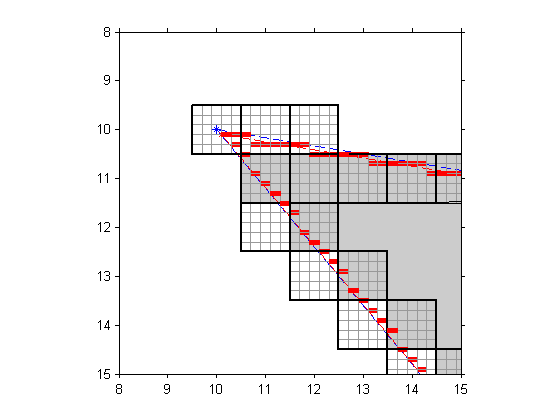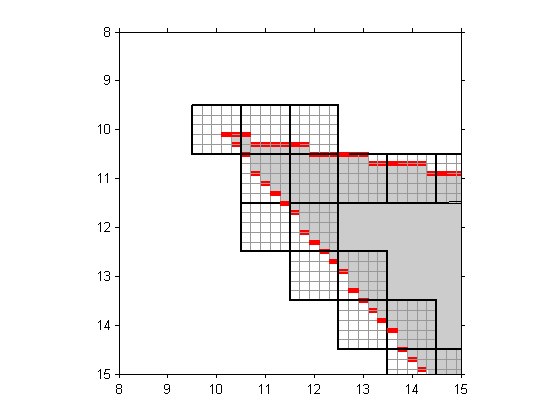# Antialiased polygon scan conversion: Are you interested?

In December I wrote several blogs about the algorithm underlying the poly2mask function. Basically, the algorithm uses a 5-by-5 subpixel grid and lays out the polygon along the edges of the grid, like this:The algorithm then makes a binary decision (inside or outside) for each pixel. A given pixel is inside the polygon if and only if the center subpixel square is inside the polygon.

A very similar procedure could be used to compute an approximate fractional coverage for each pixel. In other words, approximately what fraction of each pixel is inside the polygon? It might look like this:For each pixel, you just divide the number of gray squares by 25 to get the approximate fractional coverage. This computation could be used to do antialiased polygon scan conversion. For example, compare the left and right images below. The left image is a binary image containing the output of poly2mask. You can see the "jaggies" along the edges. The right image is a grayscale image containing the fractional coverage of each pixel. The edges in this image appear smoother.Readers: Would you like to see this functionality in the Image Processing Toolbox? If so, what do you think you might use it for?

Published with MATLAB® 7.3

|